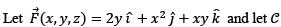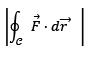#be the curve of intersection of the plane x + y + z = 1 and the cylinder x2 + y2 = 1. Then the value ofisa)πb)2πc)3π/2d)3πCorrect answer is option 'B'. Can you explain this answer? Related Test: Math - 2019 Past Year Paper

## IIT JAM QuestionAsf Institute 2 days ago